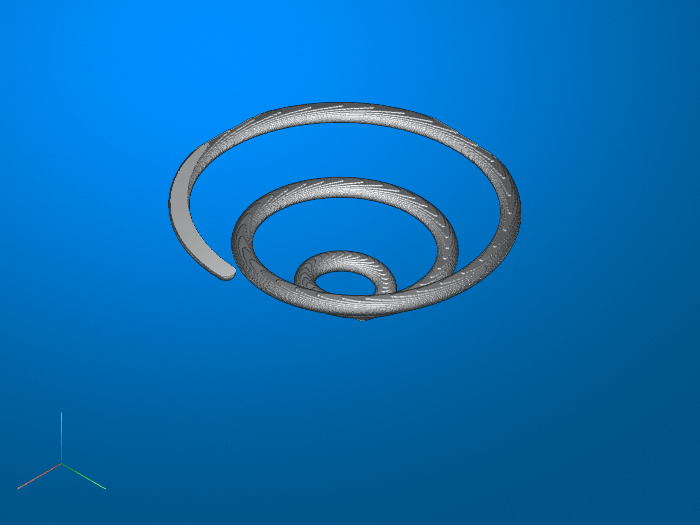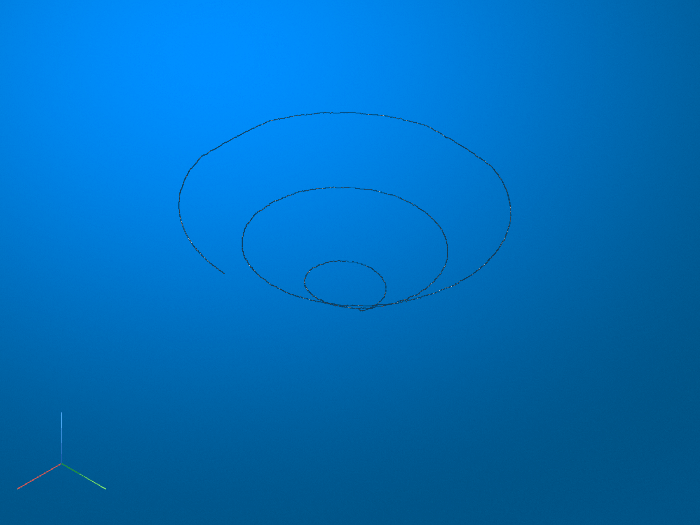Documentation

# bwskel

Reduce all objects to lines in 2-D binary image or 3-D binary volume

## Syntax

``B = bwskel(A)``
``B = bwskel(V)``
``B = bwskel(___,'MinBranchLength',N)``

## Description

example

````B = bwskel(A)` reduces all objects in the 2-D binary image `A` to 1-pixel wide curved lines, without changing the essential structure of the image. This process, called skeletonization, extracts the centerline while preserving the topology and Euler number of the objects.```

example

````B = bwskel(V)` returns the skeleton of a 3-D binary volume.```

example

````B = bwskel(___,'MinBranchLength',N)` specifies the minimum branch length `N` of the skeleton. `bwskel` removes (prunes) all branches shorter than the specified length. `bwskel` calculates the length as the number of pixels in a branch using 8-connectivity for 2-D and 26-connectivity for 3-D.```

## Examples

collapse all

Read a 2-D grayscale image into the workspace. Display the image. Objects of interest are dark threads against a light background.

```I = imread('threads.png'); imshow(I)```Skeletonization requires a binary image in which foreground pixels are `1` (white) and the background is `0` (black). To make the original image suitable for skeletonization, take the complement of the image so that the objects are light and the background is dark. Then, binarize the result.

```Icomplement = imcomplement(I); BW = imbinarize(Icomplement); imshow(BW)```Perform skeletonization of the binary image using `bwskel`.

`out = bwskel(BW);`

Display the skeleton over the original image by using the `labeloverlay` function. The skeleton appears as a 1-pixel wide cyan line over the dark threads.

`imshow(labeloverlay(I,out,'Transparency',0))`Prune small spurs that appear on the skeleton and view the result. One short branch is pruned from a thread near the center of the image.

```out2 = bwskel(BW,'MinBranchLength',15); imshow(labeloverlay(I,out2,'Transparency',0))```Read a binary image into the workspace.

`BW1 = imread('circbw.tif');`

Skeletonize objects in the image using the `bwskel` function.

`BW2 = bwskel(BW1);`

View the original image and the skeletonized image side by side.

`montage({BW1,BW2},'BackgroundColor','blue','BorderSize',5)`Load a volumetric data set into the workspace. The name of the data set is `spiralVol`. Display the volume using `volshow`.

```load spiralVol.mat; volshow(spiralVol);```Convert the `spiralVol` data set to a binary format which is required by the `bwskel` function.

`spiralVolLogical = imbinarize(spiralVol);`

Skeletonize the spiral shape in the data set. Display the skeletonized volume with `volshow`.

`spiralVolSkel = bwskel(spiralVolLogical);`

.## Input Arguments

collapse all

Binary image, specified as a 2-D logical array.

Data Types: `logical`

3-D binary volume, specified as a 3-D logical array.

Data Types: `logical`

Minimum branch length, specified as a nonnegative integer. `bwskel` prunes branches shorter than `N`. By default, `bwskel` does not prune branches.

## Output Arguments

collapse all

Skeletonized image or volume, returned as a 2-D or 3-D logical array of the same size as the input image or volume.

## Tips

• While both `bwskel` and `bwmorph` can skeletonize 2-D images, you might get different results using `bwmorph` than when using `bwskel`. Because they use different algorithms, the `bwskel` function uses 4-connectivity with 2-D images; `bwmorph` uses 8-connectivity.

• `bwskel` assumes that foreground objects in the binary image are white (logical `true`). If your image has a white background and black objects, then use the complement of your image as the input to `bwskel`. You can compute the complement using `imcomplement`.

## Algorithms

• The `bwskel` function uses the medial axis transform.

 Ta-Chih Lee, Rangasami L. Kashyap and Chong-Nam Chu. Building skeleton models via 3-D medial surface/axis thinning algorithms. Computer Vision, Graphics, and Image Processing, 56(6):462-478, 1994.

 Kerschnitzki, M, Kollmannsberger, P, Burghammer, M. et al. Architecture of the osteocyte network correlates with bone material quality. Journal of Bone and Mineral Research, 28(8):1837-1845, 2013.